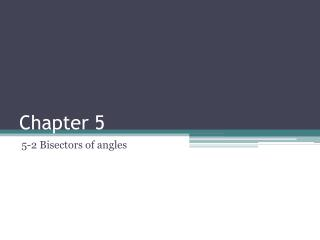# Chapter 5 - PowerPoint PPT PresentationDownload PresentationChapter 5

Chapter 5
Download Presentation## Chapter 5

- - - - - - - - - - - - - - - - - - - - - - - - - - - E N D - - - - - - - - - - - - - - - - - - - - - - - - - - -
##### Presentation Transcript

1. Chapter 5 5-2 Bisectors of angles

2. Objectives Prove and apply properties of perpendicular bisectors of a triangle. Prove and apply properties of angle bisectors of a triangle.

3. Point of concurrency • When three or more lines intersect at one point, the lines are said to be concurrent. The point of concurrency is the point where they intersect. In the construction, you saw that the three perpendicular bisectors of a triangle are concurrent. This point of concurrency is the circumcenter of the triangle.

4. Point of concurrency

5. circumcenter • The circumcenter can be inside the triangle, outside the triangle, or on the triangle.

6. circumscribed • The circumcenter of ΔABC is the center of its circumscribed circle. A circle that contains all the vertices of a polygon is circumscribed about the polygon.

7. Example 1: Using Properties of Perpendicular Bisectors • DG, EG, and FG are the perpendicular bisectors of ∆ABC. Find GC. G is the circumcenter of ∆ABC. By the Circumcenter Theorem, G is equidistant from the vertices of ∆ABC. GC = GB GC = 13.4

8. Example #2 • Use the diagram. Find GM. • MZis a perpendicular bisector of ∆GHJ. • GM = MJ • GM = 14.5

9. Example#3 • Use the diagram. Find JZ. Z is the circumcenter of ∆GHJ. By the Circumcenter Theorem, Z is equidistant from the vertices of ∆GHJ. JZ = GZ JZ = 19.9

10. Student guided practice • Do problems 3-5 in your book page 323

11. Example#4 • Find the circumcenter of ∆HJK with vertices H(0, 0), J(10, 0), and K(0, 6). • Solution: • Step 1 Graph the triangle.

12. solution • Step 2 Find equations for two perpendicular bisectors. • Since two sides of the triangle lie along the axes, use the graph to find the perpendicular bisectors of these two sides. The perpendicular bisector of HJ is x = 5, and the perpendicular bisector of HK is y = 3

13. solution • Step 3 Find the intersection of the two equations. The lines x = 5 and y = 3 intersect at (5, 3), the circumcenter of ∆HJK.

14. Example #5 • Find the circumcenter of ∆GOH with vertices G(0, –9), O(0, 0), and H(8, 0) . • Solution: • Step 1 Graph the triangle.

15. solution • Step 2 Find equations for two perpendicular bisectors. • Since two sides of the triangle lie along the axes, use the graph to find the perpendicular bisectors of these two sides. The perpendicular bisector of GO is y = –4.5, and the perpendicular bisector of OH is • x = 4.

16. Solution • Step 3 Find the intersection of the two equations • The lines x = 4 and y = –4.5 intersect at (4, –4.5), the circumcenter of ∆GOH.

17. Incenter of the triangle • A triangle has three angles, so it has three angle bisectors. The angle bisectors of a triangle are also concurrent. This point of concurrency is the incenter of the triangle.

18. Incenter of the triangles • Unlike the circumcenter, the incenter is always inside the triangle.

19. Inscribed circle • The incenter is the center of the triangle’s inscribed circle. A circle inscribedin a polygon intersects each line that contains a side of the polygon at exactly one point.

20. Example 6 : Using Properties of Angle Bisectors • MP and LP are angle bisectors of ∆LMN. Find the distance from P to MN. P is the incenter of ∆LMN. By the Incenter Theorem, P is equidistant from the sides of ∆LMN. The distance from P to LM is 5. So the distance from P to MN is also 5.

21. Example#7 • MP and LP are angle bisectors of ∆LMN. Find mPMN.

22. Student guided practice • Do problems 7-10 in your book page 323

23. Homework • 12-19 in your book page 232

24. Closure • Today we learned about bisectors of angels • Next class we are going to learned about Medians and altitudes of triangles.